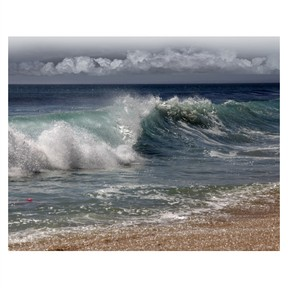Introduction to waves

# Introduction to waves

I can describe the motion and types of waves.

No account needed.8,000 schools use Gynzy92,000 teachers use Gynzy1,600,000 students use Gynzy

## General

Students are introduced to waves and are shown examples of types of waves as well as how to measure amplitude.

NGSS: MS-PS4-1

## Learning objective

Students will be able to describe the motion and types of waves.

## Introduction

Start the lesson with an introduction experiment. Follow the instructions given on the interactive whiteboard. Have students work in pairs to answer the questions given about the movement of the rope.

## Instruction

Introduce students to waves. Explain that waves in different forms are all around us, and are much more than the waves in the water that they may be familiar with. Define waves as being a disturbance that travels through matter and that they carry and transmit energy. Ask students if they can come up with examples of waves around us, and show the images on the interactive whiteboard as a selection of common waves that students might come up with. Then discuss the movement of waves by discussing the rope you started with. Show that a wave moves through markers but that they return to their exact same place after the wave moves through them. Discuss the distinction between energy and matter and show that this proves that waves carry energy but do not carry matter, as the energy moves the rope, but after it passes the matter stays in the same place. Return to the rope on the doorknob and demonstrate a small shake and a bigt shake. Have students discuss the result with their partner. Then as a class you should discuss that the amount of energy used is directly related to how big the wave is. More energy creates larger waves. Discuss with students that you can determine how large a wave is by measuring its amplitude. Then introduce the two types of waves: transverse and longitudinal and give examples of each kind. Next discuss the fact that many waves travel through a medium- or matter particles that vibrate as a wave passes through it.

## Quiz

Students first answer true/false questions to check their knowledge of key concepts introduced during the lesson. They then answer multiple-choice questions about wave types, size of waves and which waves are stronger.

## Closing

Repeat the learning goal of being able to describe wave types and motion and discuss some of the waves in the world around us. To close the lesson, ask students to answer the last two questions on the interactive whiteboard.

## Instruction materials

- Rope (about 3 feet long)

### The online teaching platform for interactive whiteboards and displays in schools

• Save time building lessons

• Manage the classroom more efficiently

• Increase student engagement